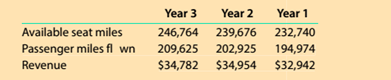Chapter 13, Problem 13.6.1MBA

Chapter
Section
Textbook Problem

Utilization rate Delta Air Lines (DAL) reported the following data (in millions) for three recent years.Delta refers to its utilization rates as passenger load factor. Compute the passenger load factor (utilization rate) for each year.

To determine

Concept Introduction:

Standard Costing System:

Standard Costing system allows estimating the costs, preparing budgets for future periods, and analyzing the performance by comparing the budgets with actual results and find variances.

To Calculate:

The utilization rate for each year

Explanation

The utilization rate for each year is calculated as follows:

 Year 3 Year 2 Year 1 Available Seat Miles (A) 246,764 239,...

Still sussing out bartleby?

Check out a sample textbook solution.

See a sample solution

The Solution to Your Study Problems

Bartleby provides explanations to thousands of textbook problems written by our experts, many with advanced degrees!

Get Started

Find more solutions based on key concepts